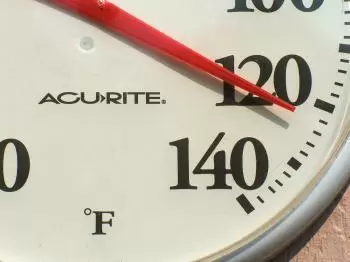# Convert 40 Degrees Celsius to Fahrenheit - Fahrenheit ScaleThe Fahrenheit scale is a temperature scale used in Anglo-Saxon countries, including the United States, Canada, and Great Britain.

This system of temperatures was proposed by the American physicist Daniel Gabriel Fahrenheit (1686-1736). Daniel Gabriel Fahrenheit also invented the thermometer, which is an instrument used to measure the temperature of a body.

However, the Fahrenheit degree is not a unit of the International Metric System of Units (SI). Twenty years later, Anders Celsius developed the centigrade scale, which nowadays is known as the Celsius scale. The degree Celsius and kelvin are the two official units in the SI.

## How to Convert 40 Degrees Celsius to Fahrenheit: Formulas and Example

We can convert degrees Celsius to Fahrenheit: we must multiply the value by 1.8 and add 32. For example, 40 degrees Celsius are 104 Fahrenheit.

On the contrary, to convert a given Fahrenheit temperature to its corresponding Celsius value, subtract 32 from the Fahrenheit temperature and then multiply by five and divide by 9.

The formulas for both conversions are as follows:

°F = °C⋅ (9/5) +32

°C = (°F -32) ⋅ 5/9

## How to Convert Degrees Fahrenheit to Kelvin?

To go from the Fahrenheit scale to the Kelvin scale, we must add 459.67, multiply by five, and divide by 9. In the same way, to go from Kelvin to Fahrenheit, we must multiply by 1.8 and subtract 459.67 from the result.

For example, the average human body temperature is set around 98.6°F. To this temperature, we add 459.67, multiply by five and divide by nine, and we obtain that the temperature of the human body in kelvin is 310.15 K.

The conversion formulas between degrees Fahrenheit and kelvins are:

°F = K ⋅ (9/5) -459.67

k = (°F +459.67) ⋅ 5/9

## How Was the Fahrenheit Scale Established?

This scale is based on two fixed points of temperature: the melting point of ice and the boiling point of water. This scale considers the freezing temperature of water at 32 degrees, and the boiling temperature of water at 212 degrees. It means that there are 180 degrees (°F) between the freezing point of water and the boiling point.

A difference of 1.8ºF is equivalent to that of 1 degree Celsius. Fahrenheit established the zero temperature (0°F), the freezing point of a 50/50 mixture of salt (ammonium chloride) and ice, and at 96°F, he took the temperature of blood (he used that of horses ).

The value 96 may seem strange, but in principle, the scale only contained twelve equal subdivisions. So Fahrenheit divided each division into eight more, obtaining 96 divisions on his scale.

This definition method is similar to that used in the Celsius scale but uses the melting and boiling points of ice and water. The Celsius scale sets these points at 0 and 100 degrees, respectively.

The Fahrenheit scale has absolute zero located at -459.67 °F. To facilitate its scientific use, the Rankine scale was created. The Rankine scale takes the 0 of the Fahrenheit scale to absolute zero, similar to what happens between the Kelvin and Celsius scales.

Author:

Published: November 7, 2017
Last review: October 10, 2022